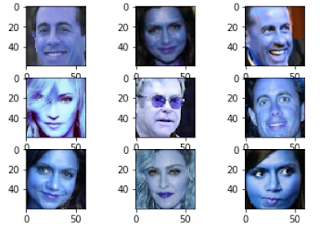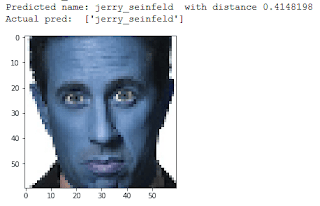### Triplet Loss - Face recognition using Keras

Face recognition is a technology capable of identifying or verifying a person from a digital image or a video frame from a video source. So in this post, we will learn how to make a face recognition system using Python and Keras. Keras is one of the simplest deep learning frameworks which helps us create neural networks. You can also check the GitHub repo for this.

## How we will do it?

Firstly we will detect the faces of people in an image for which we will be using Haar Cascades in the OpenCV package. Now there are two methods through which we can create our model. One is by simply detecting the categories of names and the other method is using Triplet loss (I will explain both of this in the coding sections)

## Coding

### Get the data

You can download the data-set here. This data set contain photos of 5 celebrity ('ben_afflek', 'elton_john', 'jerry_seinfeld', 'madonna', 'mindy_kaling').

### Preparing the dataset

Now after you have downloaded the dataset we can take out photos of faces of each celebrity to be used in our model

``````face_cascade = cv2.CascadeClassifier('haar.xml')
dirs = "data/train/"
img_size = 60

data = []
for name in os.listdir(dirs):
for f in os.listdir(dirs+name):
for x,y,w,h in faces:
img = f[y:y+h, x:x+w]
img = cv2.resize(img, (img_size,img_size))
data.append((img, name))

df = pd.DataFrame(data, columns=["image", "name"])
print("Length:",len(df))``````

we can use the same method to get the validation images also. We can check our data.

``````idx = 34
row = df.iloc[idx, :]
print("Name: ", row["name"])
plt.imshow(row.image)``````Don't worry about the blue tint, sometime OpenCV images do that. Now that we have got the faces out, we can have the convert names to integers values. I am using LabelEncoder for this.

``````le = LabelEncoder()
le.fit(df["name"].values)``````

Prepare the training set for fitting to the model.

``````x_train = list(df.image.values)
x_train = np.array(x_train)/255
y_train = le.transform(df["name"].values)``````

### Build and Fit the Model

We can do this in two ways.

#### Normal method

Using this method we fit the data in a model and try to predict the category/name of the person to whom it may belong. For this, we will be using categorical loss. Firstly let's prepare the model. We have used convolutional layers to reduce the number of parameters.

``````def get_model():
model = tf.keras.Sequential()

model.summary()
return model``````

Now that our model is ready we can feed our images to it and train it.

``````model = get_model()
model.fit(x_train,y_train,epochs=50, batch_size=100, callbacks=[checkpoint])``````

Now, this was the basic or traditional way of doing this. There is another way to do face recognition.

#### Using Triplet Loss

Triplet Loss is a concept in which we compare an image to its same (positive) type and different (negative) type. Basically, we train our model to differentiate between the same person and the different person. We encode an image to the k number vector. Then we can use this encoding to differentiate between different persons. After creating the encodings we can either use a machine-learning algorithm like k-means or simply calculate the distance between the encoding and encoding of the different persons to get the shortest distance. So first let's create a loss function in Tensorflow to check the triplet loss.

``````def triplet_loss(y_true, y_pred, alpha = 0.2):
total_lenght = y_pred.shape.as_list()[-1]
anchor, positive, negative = y_pred[:,:int(1/3*total_lenght)], y_pred[:,int(1/3*total_lenght):int(2/3*total_lenght)], y_pred[:,int(2/3*total_lenght):]
pos_dist = tf.reduce_sum(tf.square(anchor - positive), axis=-1)
neg_dist = tf.reduce_sum(tf.square(anchor - negative), axis=-1)
basic_loss = pos_dist - neg_dist + alpha
loss = tf.reduce_sum(tf.maximum(basic_loss,0.0))
return loss``````

Now that we have created a loss function we can create generate the data for triplet loss. This function will return triplets of anchor image, positive image, and negative image.

``````def generate_triplets(x, y, num_same = 4, num_diff = 4):
anchor_images = np.array([]).reshape((-1,)+ x.shape[1:])
same_images = np.array([]).reshape((-1,)+ x.shape[1:])
diff_images = np.array([]).reshape((-1,)+ x.shape[1:])

for i in range(len(y)):
point = y[i]
anchor = x[i]
same_pairs = np.where(y == point)
same_pairs = np.delete(same_pairs , np.where(same_pairs == i))
diff_pairs = np.where(y != point)

same = x[np.random.choice(same_pairs,num_same)]
diff = x[np.random.choice(diff_pairs,num_diff)]

anchor_images = np.concatenate((anchor_images, np.tile(anchor, (num_same * num_diff, 1, 1, 1) )), axis = 0)

for s in same:
same_images = np.concatenate((same_images, np.tile(s, (num_same, 1, 1, 1) )), axis = 0)

diff_images = np.concatenate((diff_images, np.tile(diff, (num_diff, 1, 1, 1) )), axis = 0)

return anchor_images, same_images, diff_images``````

Now let's create the model.

```def get_model():
model = tf.keras.Sequential()
model.summary()
return model```

Now let's create the necessary inputs and data for the triplet loss model.

``````anchor_images, same_images, diff_images = generate_triplets(x_train,y_train, num_same= 10, num_diff=10)
print(anchor_images.shape, same_images.shape, diff_images.shape)``` `````````anchor_input = tf.keras.layers.Input((img_size, img_size, 3), name='anchor_input')
positive_input = tf.keras.layers.Input((img_size, img_size, 3), name='positive_input')
negative_input = tf.keras.layers.Input((img_size, img_size, 3), name='negative_input')

shared_dnn = get_model()
encoded_anchor = shared_dnn(anchor_input)
encoded_positive = shared_dnn(positive_input)
encoded_negative = shared_dnn(negative_input)

merged_vector = tf.keras.layers.concatenate([encoded_anchor, encoded_positive, encoded_negative], axis=-1, name='merged_layer')

model = tf.keras.Model(inputs=[anchor_input,positive_input, negative_input], outputs=merged_vector)
model.summary()

anchor_model = tf.keras.Model(inputs = anchor_input, outputs=encoded_anchor)``````

Now that we have created the model and data. Let's train our model

``````Y_dummy = np.empty((anchor_images.shape,1))
model.fit([anchor_images,same_images,diff_images],y=Y_dummy, batch_size=128, epochs=100)``````

To show the encoding on a scatter plot lets create a PCA plot.

``````pca = PCA(n_components=2)
pred_pca = pca.fit_transform(pred)
plt.scatter(pred_pca[:,0], pred_pca[:,1], c=y_train)``````
````

We can see different groups of different persons. To detect the person lets create a dictionary of person encoding and the function to check the test images

```def encode_image(model ,img):
encode = model.predict(img.reshape((1,)+ img.shape))
return encode

def dist_imgs(anchor_enc, img_enc):
return np.linalg.norm(img_enc - anchor_enc)

def predict_image(model, img, dictionary):
enc = encode_image(model, img)
max_dist = 10000000
max_name = None
for name in dictionary:
dist = dist_imgs(dictionary[name], enc)
print("Name: ", name, "Dist: ", dist)
if max_dist > dist:
max_dist = dist
max_name = name
return max_name, max_dist

name_dict = {}
for i in set(df["name"].values):
img = df[df["name"] == i].iloc[0,0]/255
enc = encode_image(anchor_model, img)
name_dict[i] = enc ```

Now let's check the code on a sample image

``````idx = 25
img = x_train[idx]
plt.imshow(img)
n, d = predict_image(anchor_model, img, name_dict)
print("Predicted name:",n ," with distance", d)
print("Actual pred: ", le.inverse_transform(y_train[idx:idx+1]))``````As expected it is detected right. You can check the whole code here. Thank you for reading this. Hope this helps.